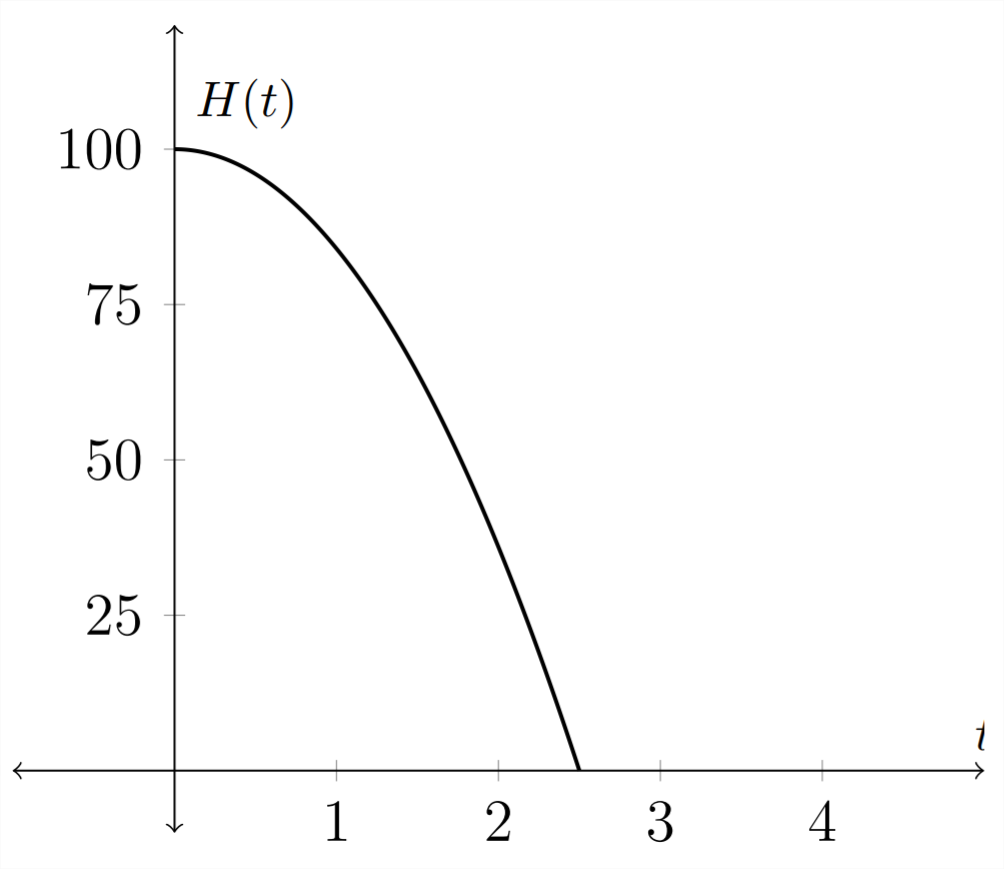# 4.8: Inverse Functions

$$\newcommand{\vecs}{\overset { \rightharpoonup} {\mathbf{#1}} }$$ $$\newcommand{\vecd}{\overset{-\!-\!\rightharpoonup}{\vphantom{a}\smash {#1}}}$$$$\newcommand{\id}{\mathrm{id}}$$ $$\newcommand{\Span}{\mathrm{span}}$$ $$\newcommand{\kernel}{\mathrm{null}\,}$$ $$\newcommand{\range}{\mathrm{range}\,}$$ $$\newcommand{\RealPart}{\mathrm{Re}}$$ $$\newcommand{\ImaginaryPart}{\mathrm{Im}}$$ $$\newcommand{\Argument}{\mathrm{Arg}}$$ $$\newcommand{\norm}{\| #1 \|}$$ $$\newcommand{\inner}{\langle #1, #2 \rangle}$$ $$\newcommand{\Span}{\mathrm{span}}$$ $$\newcommand{\id}{\mathrm{id}}$$ $$\newcommand{\Span}{\mathrm{span}}$$ $$\newcommand{\kernel}{\mathrm{null}\,}$$ $$\newcommand{\range}{\mathrm{range}\,}$$ $$\newcommand{\RealPart}{\mathrm{Re}}$$ $$\newcommand{\ImaginaryPart}{\mathrm{Im}}$$ $$\newcommand{\Argument}{\mathrm{Arg}}$$ $$\newcommand{\norm}{\| #1 \|}$$ $$\newcommand{\inner}{\langle #1, #2 \rangle}$$ $$\newcommand{\Span}{\mathrm{span}}$$$$\newcommand{\AA}{\unicode[.8,0]{x212B}}$$

An inverse function undoes the action of the original function. So the inverse of a function that squared a number would be a function that square rooted a number. In general, an inverse function will take a $$y$$ value from the original function and return the $$x$$ value that produced it.

We can see this in an application. Given an object with little or no air resistance that is dropped from $$100 \mathrm{ft}$$, the function that describes its height as a function of time would be:
$H(t)=100-16 t^{2}$
In this function, $$H(t)$$ is the height of the object at time $$t .$$ If we wanted to turn this around so that it described the time for a given height, then we would want to isolate the $$t$$ variable. In this example, the graph of the function would change in that the original independent variable - $$t,$$ becomes the dependent variable in the inverse function.
\begin{aligned} h &=100-16 t^{2} \\ 16 t^{2} &=100-h \\ t^{2} &=\frac{100-h}{16} \\ t &=\sqrt{\frac{100-h}{16}} \\ T(h) &=\frac{\sqrt{100-h}}{4} \end{aligned}

Original function: $$H(t)=100-16 t^{2}$$Inverse function: $$T(h)=\frac{\sqrt{100-h}}{4}$$Notice that the graph of the function inverse is the original function reflected over the line $$y=x,$$ because an inverse function interchanges the independent and dependent variables.

Finding a formula for an inverse function can be more confusing when we consider a standard function $$y=f(x) .$$ In our standard notation, $$x$$ is always considered to be the independent variable and $$y$$ is always considered to be the dependent variable.

Notice in the example above that when we graphed the function and its inverse, the label on the $$x$$ axis changed from $$t$$ to $$h .$$ In a standard function, the $$x$$ axis will always be the $$x$$ axis and the $$y$$ axis will always be the $$y$$ axis. To compensate for this, when we find a function inverse for a function stated in terms of $$x$$ and $$y$$ we generally interchange the $$x$$ and $$y$$ terms so that $$x$$ remains the independent variable.
$H(t)=100-16 t^{2}$
and found the inverse to be
$T(h)=\frac{\sqrt{100-h}}{4}$
If the original function had been stated in terms of $$x$$ and $$y,$$ then the process would have looked like this:
\begin{aligned}
f(x) &=100-16 x^{2} \\
y &=100-16 x^{2} \\
16 x^{2} &=100-y \\
x^{2} &=\frac{100-y}{16} \\
x &=\sqrt{\frac{100-y}{16}} \\
x &=\frac{\sqrt{100-y}}{4}
\end{aligned}

Then we switch the $$x$$ and $$y$$ variables to keep the $$x$$ as the independent variable:
$y=f^{-1}(x)=\frac{\sqrt{100-x}}{4}$
$$\mathrm{So}$$
$f(x)=100-16 x^{2}$
and
$f^{-1}(x)=\frac{\sqrt{100-x}}{4}$

Exercises 4.8
Given the function $$f(x),$$ find the inverse function $$f^{-1}(x)$$
1) $$\quad f(x)=3 x$$
2) $$\quad f(x)=-4 x$$
3) $$\quad f(x)=4 x+2$$
4) $$\quad f(x)=1-3 x$$
5) $$\quad f(x)=x^{3}-1$$
6) $$\quad f(x)=x^{3}+1$$
7) $$\quad f(x)=x^{2}+4 (x \geq 4)$$
8) $$\quad f(x)=x^{2}+9 (x \geq 9)$$
9) $$\quad f(x)=\frac{4}{x}$$
10) $$\quad f(x)=-\frac{3}{x}$$
11) $$\quad f(x)=\frac{1}{x-2}$$
12) $$\quad f(x)=\frac{4}{x+2}$$
13) $$\quad f(x)=\frac{2}{x+3}$$
14) $$\quad f(x)=\frac{4}{2-x}$$
15) $$\quad f(x)=\frac{3 x}{x+2}$$
16) $$\quad f(x)=-\frac{2 x}{x-1}$$
17) $$\quad f(x)=\frac{2 x}{3 x-1}$$
18) $$\quad f(x)=-\frac{3 x+1}{x}$$
19) $$\quad f(x)=\frac{3 x+4}{2 x-3}$$
20) $$\quad f(x)=\frac{2 x-3}{x+4}$$
21) $$\quad f(x)=\frac{2 x+3}{x+2}$$
22) $$\quad f(x)=-\frac{3 x+4}{x-2}$$
Find the inverse function for each of the following applications.
23) The volume of water left in a 1000 gallon tank that drains in 40 minutes is modeled by the equation:
$V(t)=1000\left(1-\frac{t}{40}\right)^{2}$
Find $$T(v)$$ - the function that tells you how long the water has been draining given a particular volume left in the tank. Time is measured in minutes and the volume is measured in gallons.
24) The speed of a vehicle in miles per hour that leaves skid marks $$d$$ feet long is modeled by the equation:
$R(d)=2 \sqrt{5 d}$
Find $$D(r)$$ - the function that tells you the stopping distance for a vehicle traveling $$r$$ miles per hour.
25) The period of a pendulum of length $$\ell$$ can be expressed by the relationship:
$T(\ell)=2 \pi \sqrt{\frac{\ell}{980}}$
Find the function $$L(t)$$ that determines the length of a pendulum given its period. Here the time is measured in seconds and the length is measured in centimeters.
26) The volume of a sphere of radius $$r$$ is given by the formula:
$V(r)=\frac{4}{3} \pi r^{3}$
Find $$R(v)$$ - the function that determines the radius of a sphere given its volume.

4.8: Inverse Functions is shared under a not declared license and was authored, remixed, and/or curated by LibreTexts.